# BST 定义

• 所有 key 小于 V 的都被存储在 V 的左子树
• 所有 key 大于 V 的都存储在 V 的右子树class BSTNode(object):
def __init__(self, key, value, left=None, right=None):
self.key, self.value, self.left, self.right = key, value, left, right


# 构造一个 BST

class BST(object):
def __init__(self, root=None):
self.root = root

@classmethod
def build_from(cls, node_list):
cls.size = 0
key_to_node_dict = {}
for node_dict in node_list:
key = node_dict['key']
key_to_node_dict[key] = BSTNode(key, value=key)   # 这里值暂时用 和 key一样的

for node_dict in node_list:
key = node_dict['key']
node = key_to_node_dict[key]
if node_dict['is_root']:
root = node
node.left = key_to_node_dict.get(node_dict['left'])
node.right = key_to_node_dict.get(node_dict['right'])
cls.size += 1
return cls(root)

NODE_LIST = [
{'key': 60, 'left': 12, 'right': 90, 'is_root': True},
{'key': 12, 'left': 4, 'right': 41, 'is_root': False},
{'key': 4, 'left': 1, 'right': None, 'is_root': False},
{'key': 1, 'left': None, 'right': None, 'is_root': False},
{'key': 41, 'left': 29, 'right': None, 'is_root': False},
{'key': 29, 'left': 23, 'right': 37, 'is_root': False},
{'key': 23, 'left': None, 'right': None, 'is_root': False},
{'key': 37, 'left': None, 'right': None, 'is_root': False},
{'key': 90, 'left': 71, 'right': 100, 'is_root': False},
{'key': 71, 'left': None, 'right': 84, 'is_root': False},
{'key': 100, 'left': None, 'right': None, 'is_root': False},
{'key': 84, 'left': None, 'right': None, 'is_root': False},
]
bst = BST.build_from(NODE_LIST)


# BST 操作

## 查找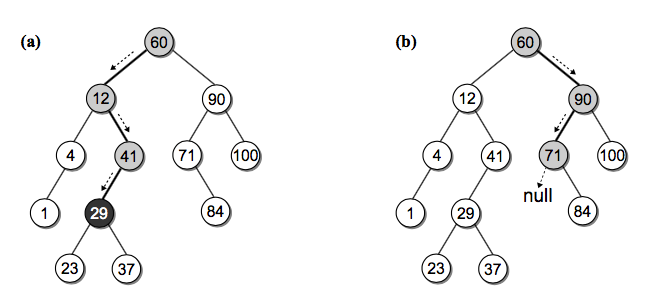def _bst_search(self, subtree, key):
if subtree is None:   # 没找到
return None
elif key < subtree.key:
return self._bst_search(subtree.left, key)
elif key > subtree.key:
return self._bst_search(subtree.right, key)
else:
return subtree

def get(self, key, default=None):
node = self._bst_search(self.root, key)
if node is None:
return default
else:
return node.value


## 获取最大和最小 key 的节点

    def _bst_min_node(self, subtree):
if subtree is None:
return None
elif subtree.left is None:   # 找到左子树的头
return subtree
else:
return self._bst_min_node(subtree.left)

def bst_min(self):
node = self._bst_min_node(self.root)
return node.value if node else None


## 插入def _bst_insert(self, subtree, key, value):
""" 插入并且返回根节点

:param subtree:
:param key:
:param value:
"""
if subtree is None:   # 插入的节点一定是根节点，包括 root 为空的情况
subtree = BSTNode(key, value)
elif key < subtree.key:
subtree.left = self._bst_insert(subtree.left, key, value)
elif key > subtree.key:
subtree.right = self._bst_insert(subtree.right, key, value)
return subtree

node = self._bst_search(self.root, key)
if node is not None:   # 更新已经存在的 key
node.value = value
return False
else:
self.root = self._bst_insert(self.root, key, value)
self.size += 1
return True


## 删除节点

• 节点是叶节点
• 节点有一个孩子
• 节点有两个孩子

#### 删除叶节点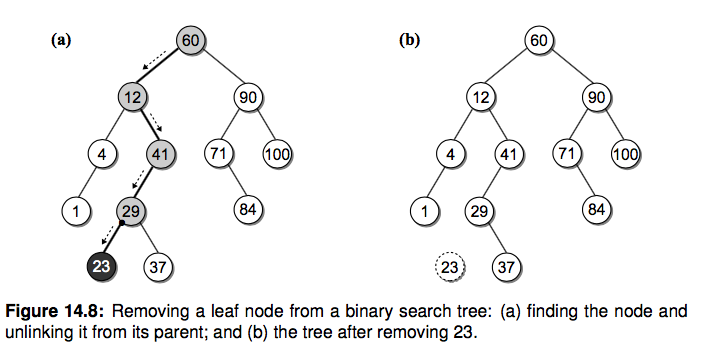#### 删除只有一个孩子的节点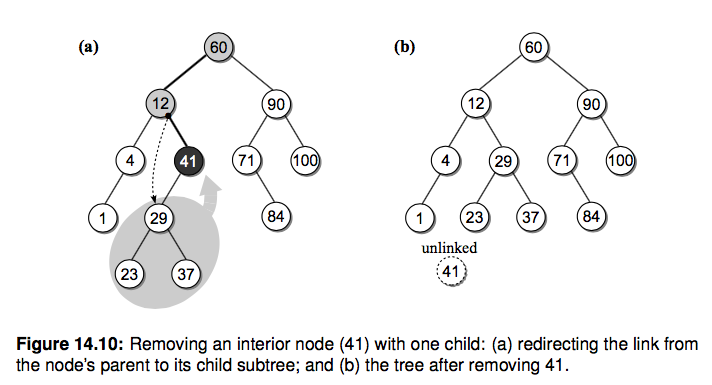#### 删除有两个孩子的内部节点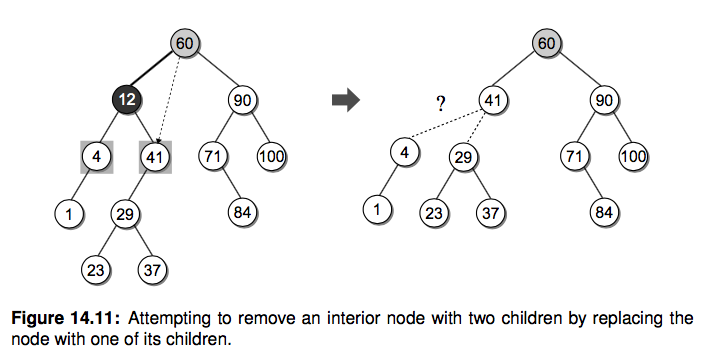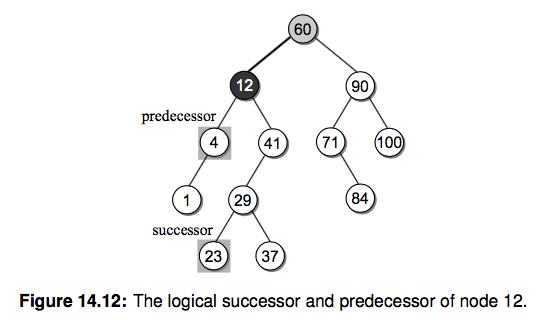12 在中序遍历中的逻辑前任和后继分别是 4 和 23 节点。于是我们还有一种方法来删除 12 这个节点：

• 找到待删除节点 N(12) 的后继节点 S(23)
• 复制节点 S 到节点 N
• 从 N 的右子树中删除节点 S，并更新其删除后继节点后的右子树def _bst_remove(self, subtree, key):
"""删除节点并返回根节点"""
if subtree is None:
return None
elif key < subtree.key:
subtree.left = self._bst_remove(subtree.left, key)
return subtree
elif key > subtree.key:
subtree.right = self._bst_remove(subtree.right, key)
return subtree
else:  # 找到了需要删除的节点
if subtree.left is None and subtree.right is None:    # 叶节点，返回 None 把其父亲指向它的指针置为 None
return None
elif subtree.left is None or subtree.right is None:  # 只有一个孩子
if subtree.left is not None:
return subtree.left   # 返回它的孩子并让它的父亲指过去
else:
return subtree.right
else:  # 俩孩子，寻找后继节点替换，并从待删节点的右子树中删除后继节点
successor_node = self._bst_min_node(subtree.right)
subtree.key, subtree.value = successor_node.key, successor_node.value
subtree.right = self._bst_remove(subtree.right, successor_node.key)
return subtree

def remove(self, key):
assert key in self
self.size -= 1
return self._bst_remove(self.root, key)


# 时间复杂度分析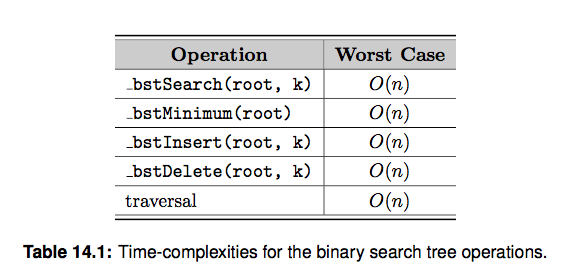# 练习题：

• 请你实现查找 BST 最大值的函数

# 延伸阅读

• 《Data Structures and Algorithms in Python》14 章，树的概念和算法还有很多，我们这里介绍最基本的帮你打个基础
• 了解红黑树。普通二叉查找树有个很大的问题就是难以保证树的平衡，极端情况下某些节点可能会非常深，导致查找复杂度大幅退化。而平衡二叉树就是为了解决这个问题。请搜索对应资料了解下。
• 了解 mysql 索引使用的 B-Tree 结构(多路平衡查找树)，这个是后端面试数据库的常考点。想想为什么？当元素非常多的时候，二叉树的深度会很深，导致多次磁盘查找。从B树、B+树、B*树谈到R 树